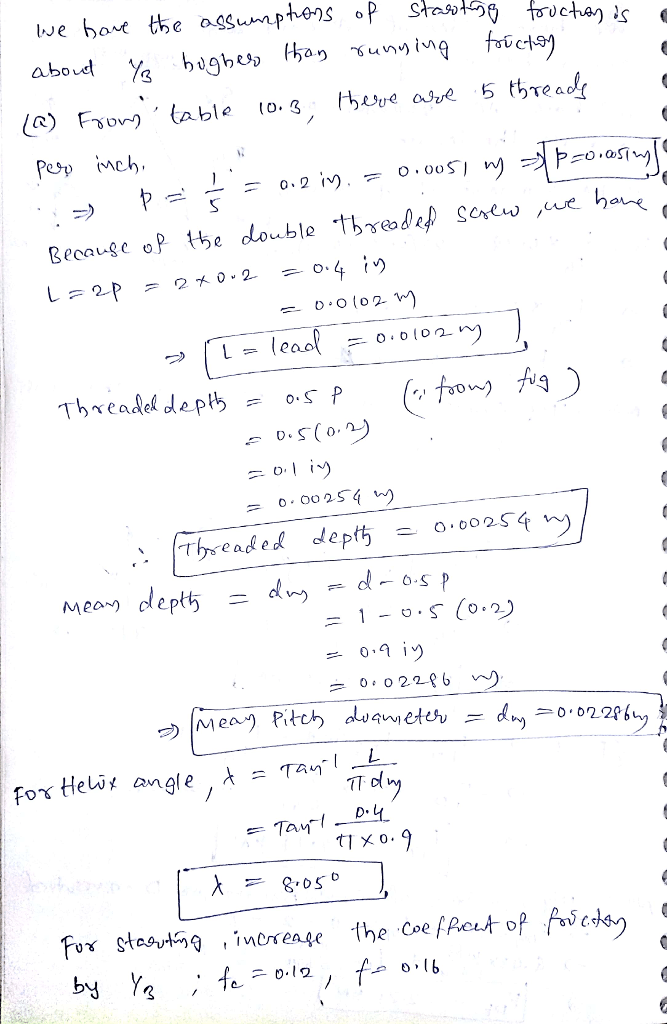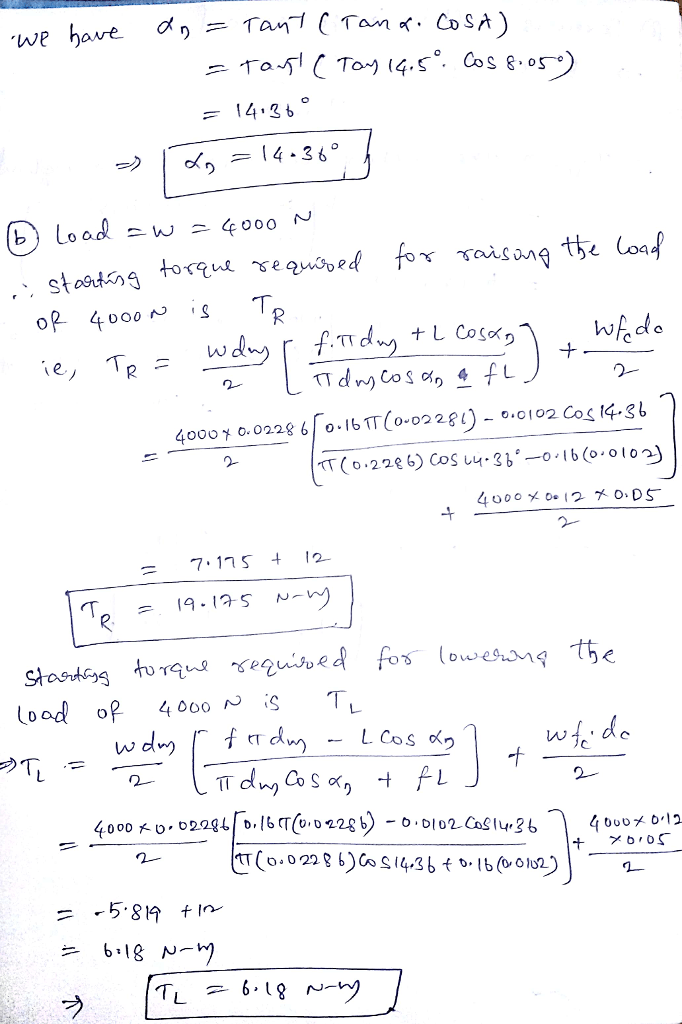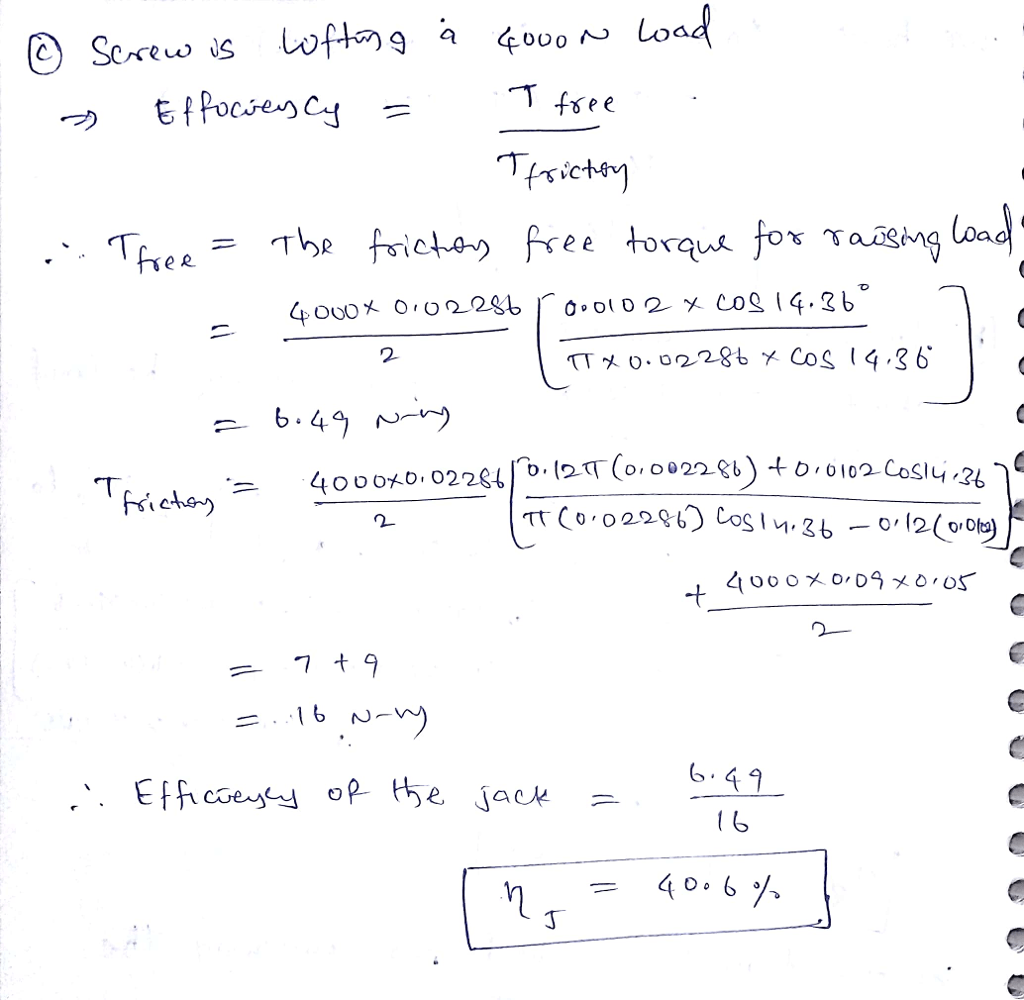# A double-threaded Acme screw of known major diameter is used in a jack having a plain thrust collar of known mean diameter. Coefficients of running friction are estimated as 0.09 for the collar and 0.12 for the screw. Assume that the starting friction is about 1/3 higher than running friction (a)Determine the pitch, lead, thread depth, mean pitch diameter, and helix angle of the screw (b)Estimate the starting torque for raising and for lowering a 4000 N load (c) lf the screw is lifting a 4000 N load, determine the efficiency of the jack Double-threaded

Question-AnswerCategory: Machine DesignA double-threaded Acme screw of known major diameter is used in a jack having a plain thrust collar of known mean diameter. Coefficients of running friction are estimated as 0.09 for the collar and 0.12 for the screw. Assume that the starting friction is about 1/3 higher than running friction (a)Determine the pitch, lead, thread depth, mean pitch diameter, and helix angle of the screw (b)Estimate the starting torque for raising and for lowering a 4000 N load (c) lf the screw is lifting a 4000 N load, determine the efficiency of the jack Double-threaded

A double-threaded Acme screw of known major diameter is used in a jack having a plain thrust collar of known mean diameter. Coefficients of running friction are estimated as 0.09 for the collar and 0.12 for the screw. Assume that the starting friction is about 1/3 higher than running friction (a)Determine the pitch, lead, thread depth, mean pitch diameter, and helix angle of the screw (b)Estimate the starting torque for raising and for lowering a 4000 N load (c) lf the screw is lifting a 4000 N load, determine the efficiency of the jack Double-threaded